Courses

# Types of Bonding Class 11 Notes | EduRev

## : Types of Bonding Class 11 Notes | EduRev

The document Types of Bonding Class 11 Notes | EduRev is a part of the Course Chemistry Class 11.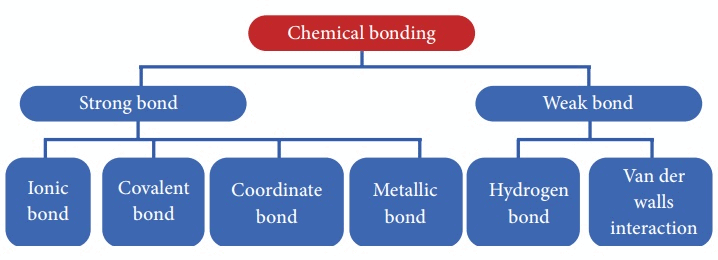When substances participate in chemical bonding and yield compounds, the stability of the resulting compound can be gauged by the type of chemical bonds it contains.
The type of chemical bonds formed vary in strength and properties.
There are 4 primary types of chemical bonds which are formed by atoms or molecules to yield compounds. These types of chemical bonds include:

• Ionic Bonds
• Covalent Bonds
• Hydrogen Bonds
• Polar Bonds

These types of bonds in chemical bonding are formed from the loss, gain, or sharing of electrons between two atoms/molecules.

Ionic Bond

When a bond is formed by complete transference of electrons from one atom to another so as to complete their outermost orbits by acquiring 8 electrons (i.e., octet) or 2 electrons (i.e., duplet) in case of hydrogen, lithium etc. and hence acquire the stable nearest noble gas configuration, the bond formed is called ionic bond or electrovalent bond.
Explanation of Ionic Bond
Atoms are electrically neutral. Therefore, they possess equal number of protons and electrons. On losing an electron, an atom becomes positively charged since now the number of protons exceeds the number of electrons.

A → A+ + e-

On the other hand, in case of atom, gaining the electron, the number of electrons exceeds the number of protons and thus the atom becomes negatively charged.

B + e→ B-

The oppositely charged particles formed above attract each other by electrostatic forces of attraction. The bond thus formed is known as electrovalent or ionic bond.

Example: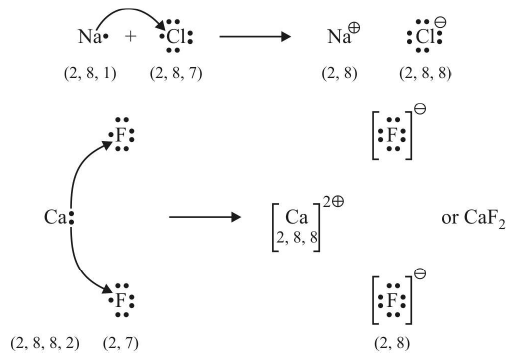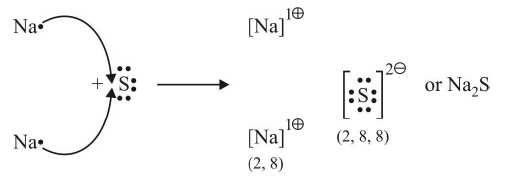Electrovalency

The number of electrons lost or gained during the formation of an electrovalent linkage is termed as the electrovalency of the element.
For example, sodium and calcium lost 1 and 2 electrons respectively and so their valencies are 1 and 2. Similarly, chlorine and oxygen gain 1 and 2 electrons respectively, so they possess an electrovalency of 1 and 2. In other words, valency is equal to the charge on the ion.

Factors governing the formation of ionic bonds
(i) Ionisation Enthalpy (Ionization Energy)
Ionisation enthaly of any element is the amount of energy required to remove an electron from the outermost shell of an isolated atom in gaseous phase so as to convert it into a gaseous positive ion.

It is clear that lesser the ionisation enthalpy, easier will be the removal of an electron, i.e., formation of a positive ion and hence greater the chances of formation of an ionic bond.

Ionisation enthalpy (I.E.) of alkali metals (i.e., group I elements) is low, hence they have more tendency to form positive ions.
(ii) Electron Gain Enthalpy (Electron Affinity)
Electron affinity or Electron gain enthalpy of an element is the enthalpy change that takes place when an extra electron is added to an isolated atom in the gaseous phase to form a gaseous negative ion.

Higher is the electron affinity, more is the energy released and stabler will be the negative ion produced. Consequently, the probability of formation of ionic bond will be enhanced.

Halogens possess high electron affinity. So the formation of their negative ions is very common, e.g., in case of chlorine, electron affinity is +348 kJ/mole, i.e.,
Cl (g) + e → Cl + 348 kJ/mole
or E.Z. = + 348 kJ mol–1
(iii) Lattice Enthalpy (Lattice Energy)
In the formation of ionic compounds, the positively charged ions combine with negatively charged ions to form the compound.

A+ (g) + B (g) → A+ B (s) The energy released when the requisite number of gaseous positive and negative ions combine to form one mole of the ionic compound is called lattice enthalpy.

Characteristics of Ionic Compounds
1. Physical State
These compounds usually exist in the solid state.

2. Crystal Structure
X-ray analysis of the ionic compounds shows that they exist as ions and not as molecules. These ions are arranged in a regular pattern in the three dimensional space to form a lattice.
The pattern of arrangement, however, depends upon the size and charges of the ions. For example, in case of sodium chloride, each sodium ion is surrounded by six chloride ions and each chloride by six sodium ions, thus giving rise to a three dimensional octahedral crystal structure (figure). The formula of an ionic compound merely indicates the relative number of ions present.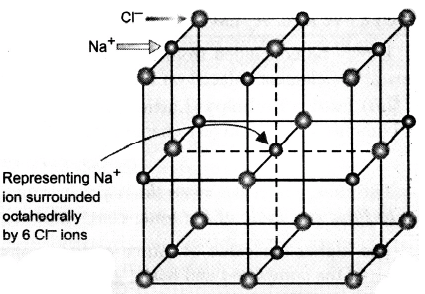Crystal Structure of NaCl

3. High melting and boiling points
Ionic compounds possess high melting and boiling points.

This is because ions are tightly held together by strong electrostatic forces of attraction and hence a huge amount of energy is required to break the crystal lattice.

4. Solubility
Electrovalent compounds are soluble in solvents like water which are polar in nature and have high dielectric constant.

It is due to the reason that the polar solvent interacts with the ions of the crystals and further the high dielectric constant of the solvent (i.e., capacity of the solvent to weaken the forces of attraction) cuts off the force of attraction between these ions. Furthermore, the ions may combine with the solvent to liberate energy called the hydration enthalpy which is sufficient to overcome the attractive forces between the ions.
Non-polar solvents like carbon tetrachloride, benzene etc. having low dielectric constants are not capable of dissolving ionic solids. Hence, ionic solids are soluble in polar solvents and insoluble in non-polar solvents.
5. Electrical conductivity
Ionic compounds are good conductors of electricity in solution or in the molten state. In solution or molten state, their ions are free to move. As the ions are charged, they are attracted towards electrodes and thus act as carriers of electric current.

6. Ionic Reactions
The reactions of the ionic compounds are, in fact, the reactions between the ions produced in solution. As the oppositely charged ions combine quickly, these reactions are, therefore, quite fast.
[e.g. Na+ Cl (aq) + Ag+ NO3 (aq) → AgCl (s) + NaNO3 (aq)]

Covalent Bond
The bond formed between the two atoms by mutual sharing of electrons between them so as to complete their octets or duplets in case of elements having only one shell is called covalent bond or covalent linkage and the number of electrons contributed by each atom is known as covalency.

Example: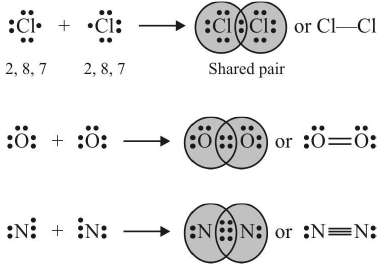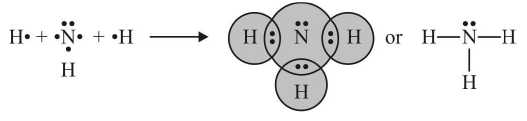Conditions for formation of covalent bonds
The following conditions favour the formation of covalent

bonds:

• Electronegativity: An atom will not transfer the electron or electrons to. the other atom if the electronegativity difference between the two. atoms is zero or very small (less than 1.6). Such atoms prefer to. share electrons, i.e., form covalent bonds.
• When both, the atoms' are short in electrons in the valency shell in comparison to. stable noble configuration, then such atoms complete the outermost shell by sharingelectrons. Except hydrogen which has one electron in valencyshell, such atoms have 5, 6 or 7 valency electrons. The non-metals of group V A, VIA and VIlA satisfy this condition.

Covalency

It is defined as the number of electrons contributed by an atom of the element for sharing with other atoms as to achieve noble gas configuration. It can also. be defined as the number of covalent bonds formed by the atom of the element with other atoms.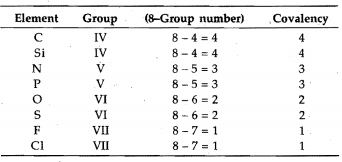Generally, the covalency of an element is equal to. the total number of unpaired electrons in s- and p-orbitals of the valency shell.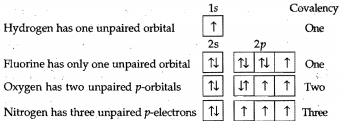These four elements do not possess d-orbitals in their valency shell. However, the elements having vacant d-orbitals in their valency shell-like P, S, CI, Br, I, show

variable covalency by increasing the number of unpaired electrons under excited conditions, i.e., unpairing the paired orbital and shifting the electrons to. vacant d-orbitals.

Example:

Draw the Lewis dot structure of HCN molecule.

Sol. Step-1: Total number of valence electrons in HCN = 1 + 4 + 5 = 10 (1H = 1, 6C = 2 ,4, 7N = 2, 5)

Step-2: Skeletal structure is HCN (C is least electronegative).
Step-3: Putting one shared pair of electrons between H and C and one between C and N, and the remaining as lone pairs, we have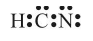In this structure, duplet of H is complete but octets of C and N are not complete. Hence, multiple bonding is required between and N. Octets of C and N will be complete if there is triple bond between C and N. Thus,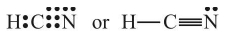Example:

Draw the Lewis dot structure of CO32– ion.

Sol. Step-1: Total number of valence electrons of CO3 = 4 + 3 × 6 = 22(6C = 2, 4, 8O = 2, 6)

Step-2: Total number of electrons to be distributed in CO32– = 22 + 2 (for two units -ve charge) = 24
Step-3: The skeletal structure of CO3 is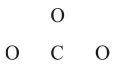Step-4: Putting one shared pair of electrons between each C and O and completing the octets of oxygen, we have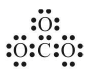In this structure, octet of C is not complete. Hence, multiple bonding is required between C and one of the O–atoms. Drawing a double bond between C and one O-atom serves the purpose: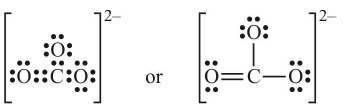Table: Lewis structures of some typical molecules and ions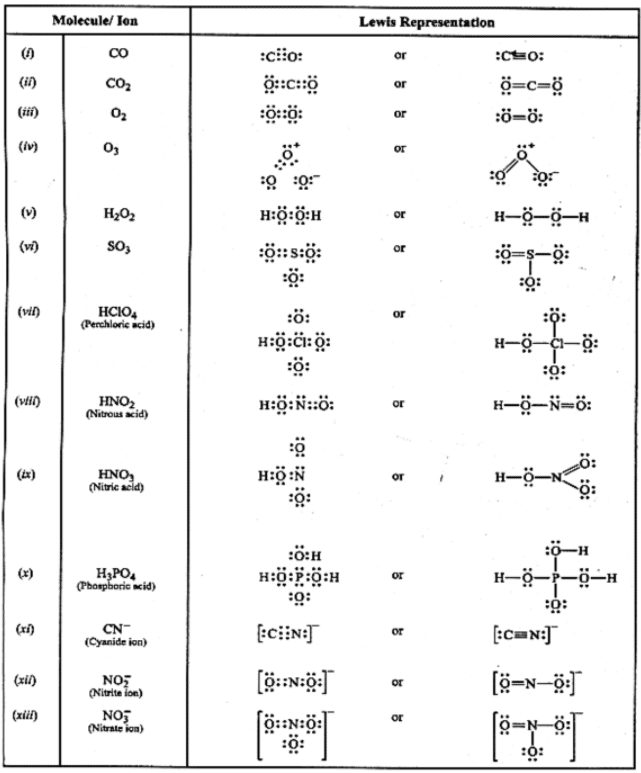Table: Lewis structures of some typical molecules and ions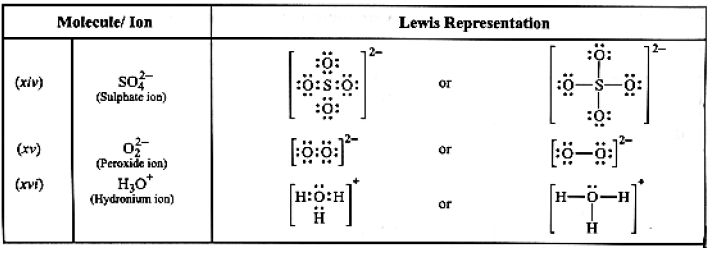Coordinate Covalent Bond or Dative Bond

• When both the electrons for sharing between two atoms are contributed by one atom only the bond formed is known as coordinate bond or dative bond. In terms of orbital theory the coordinate covalent bond is formed by overlapping between empty and completely filled atomic orbitals.
• The atom donating the pair of electrons is called donor and the atom which accepts the pair of electrons is called acceptor. The compounds containing coordinate bonds are known as coordination compounds.
• The bond is represented by an arrow () pointing head towards the acceptor.

Once the coordinate bond is formed it is indistinguishable from a covalent bond. Examples:

1. Formation of SO2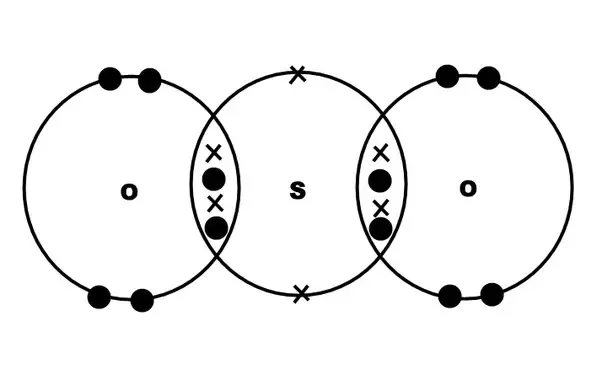2. Formation of SO3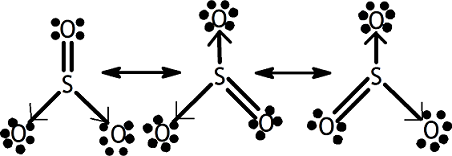3. Formation of Hydroxonium ion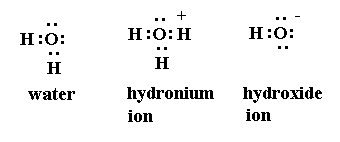4. NH3 and BF3 form addition product by Coordinate covalent bond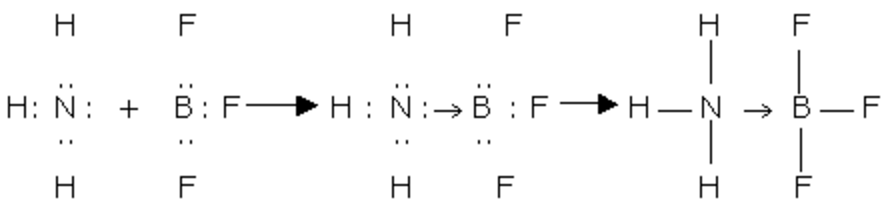Offer running on EduRev: Apply code STAYHOME200 to get INR 200 off on our premium plan EduRev Infinity!

## Chemistry Class 11

136 videos|245 docs|180 tests